# Lamé coefficients

of an orthogonal curvilinear coordinate system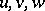in space

The quantities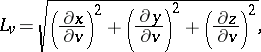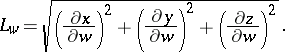The Lamé coefficients in the plane are defined similarly. In terms of the Lamé coefficients in the coordinatesone can express the element of arc length: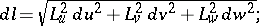the element of surface area: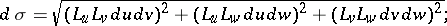and the volume element:The Lamé coefficients occur in the expressions for the operations of vector analysis in the coordinates: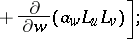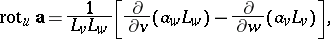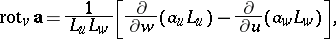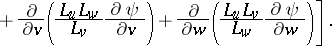For the Lamé coefficients of various orthogonal curvilinear coordinates see the corresponding articles on those coordinates.

Lamé coefficients were introduced by G. Lamé .